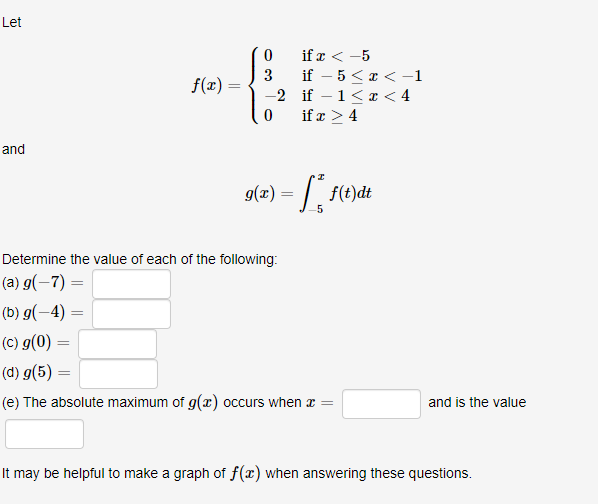# Let(0 ifx-5f(x)0 ifz 4and9(x)-f(t)dt5Determine the value of each of the following(d) g(5)(e) The absolute maximum of g(z) occurs when z-and is the valueIt may be helpful to make a graph of f(z) when answering these questions

Questionhelp_outlineImage TranscriptioncloseLet (0 ifx-5 f(x) 0 ifz 4 and 9(x)-f(t)dt 5 Determine the value of each of the following (d) g(5) (e) The absolute maximum of g(z) occurs when z- and is the value It may be helpful to make a graph of f(z) when answering these questions fullscreen
check_circleExpert Solution
Step 1

The functions are given as

Step 2

The graph of the function f (x) is obtained as follows.

Step 3

(a) To determine the value g (&ndash...

### Want to see the full answer?

See Solution

#### Want to see this answer and more?

Solutions are written by subject experts who are available 24/7. Questions are typically answered within 1 hour*

See Solution
*Response times may vary by subject and question
Tagged in

### Integration